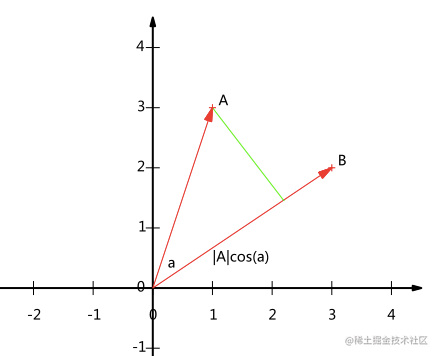# 机器学习之 PCA(主成分分析)

PCA是一种具有严格数学基础并且已被广泛采用的降维方法

### 1.向量的内积和投影我们知道，内积还可表示为，也就是说，A与B的内积等于A到B的投影长度乘以B的模

## 优化目标

### 协方差矩阵对角化

1）实对称矩阵的特征值都为实数
2）实对称矩阵的所有特征向量正交 。
3）设特征值重数为r，则必然存在r个线性无关的特征向量对应于，因此可以将这r个特征向量单位正交化。

P是协方差矩阵的特征向量单位化后按列排列出的矩阵，其中每一列都是C的一个特征向量。如果设P按照中特征值的从大到小，将特征向量从左到右排列，则用P的前K行组成的矩阵的装置乘以原始数据矩阵X，就得到了我们需要的降维后的数据矩阵Y。

### PCA算法

1）将原始数据按列组成n行m列矩阵X

2）将X的每一行（代表一个属性字段）进行零均值化，即减去这一行的均值

3）求出协方差矩阵C=\frac{1}{m}XX^\mathsf{T}

4）求出协方差矩阵的特征值及对应的特征向量

5）将特征向量按对应特征值大小从左到右按列排列成矩阵，取前k列组成矩阵P

6）Y=P^TX即为降维到k维后的数据

### 代码实现

import numpy as np
import pandas as pd

## X: 需要降维的原始数据   topNfeat：需要降到的维数
def PCA(X, topNfeat=9999999):
# 1.原始数据默认都是每一行为一个样本数据，每一列为一个特征，
#   所以进行转置，让每一列代表一个样本数据
X = X.T
# 2.将数据的每一行（代表一个属性字段）进行零均值化
meanValues = np.mean(X, axis=1) #计算每一行的均值
meanValues = np.mat(meanValues).T #将一个向量转换成n*1的矩阵
meanRemoved = X - meanValues   #均值归零

#3.求出协方差矩阵
covMat = np.cov(meanRemoved) #cov计算协方差时除的是 （样本个数-1）,也就是自由度
#     covMat =  meanRemoved @ meanRemoved.T / (meanRemoved.shape)
print("协方差矩阵：\n",covMat)

#4求出协方差矩阵的特征值及对应的特征向量
eigVals, eigVects = np.linalg.eig(covMat)  #eigVals:特征值  eigVects：特征向量
print("特征值\n",eigVals)
print("特征向量\n",eigVects)

#5将特征向量按对应特征值大小从左到右按列排列成矩阵，取前k列组成矩阵
# argsort函数返回的是数组值从小到大的索引值,参数中加个-号，变为从大到小
eigValInd = np.argsort(-eigVals)
eigValInd = eigValInd[0:topNfeat]  #取出前topNfeat个最大的特征值所对应的索引
redEigVects = eigVects[:,eigValInd]  #redEigVects 即为需要的变换矩阵，即P
print("变换矩阵：\n",redEigVects)

#6 Y=P^TX即为降维到k维后的数据
X_PCA = redEigVects.T @ X
return X_PCA

PCA(X, topNfeat = 1)

协方差矩阵：
[[1.5 1. ]
[1.  1.5]]

[2.5 0.5]

[[ 0.70710678 -0.70710678]
[ 0.70710678  0.70710678]]

[[0.70710678]
[0.70710678]]

array([[-2.12132034, -0.70710678,  0.        ,  2.12132034,  0.70710678]])

### sklearn

sklearn中为我们已经封装好了对应的PCA接口，下面我们使用PCA对sklearn中自带的一个手写数字数据集进行降维。

from sklearn import datasets

digits = datasets.load_digits()
X = digits.data
y = digits.target
X.shape,y.shape

((1797, 64), (1797,))

from sklearn.model_selection import train_test_split
X_train, X_test, y_train, y_test = train_test_split(X, y, random_state=666)
X_train.shape

(1347, 64)

%%time

from sklearn.neighbors import KNeighborsClassifier

knn_clf = KNeighborsClassifier()
knn_clf.fit(X_train, y_train)
knn_clf.score(X_test,y_test)  #准确率为

Wall time: 69.8 ms

knn_clf.score(X_test,y_test)  #准确率为

0.9866666666666667

#decomposition(分解)
from sklearn.decomposition import PCA

# n_components 要降到的维数，第一次我们直接降到2维
pca = PCA(n_components =2)
pca.fit(X_train)
X_train_reducation = pca.transform(X_train)
X_test_reducation = pca.transform(X_test) #注意测试集也需要做降维处理
X_train_reducation.shape

(1347, 2)

pca.explained_variance_ratio_
#代表降维后的各主成分的方差值占总方差值的比例，这个比例越大，则越是重要的主成分。

array([0.14566817, 0.13735469])

%%time

knn_clf = KNeighborsClassifier()
knn_clf.fit(X_train_reducation, y_train) # #降维后，所用时间明显减少

Wall time: 2 ms

knn_clf.score(X_test_reducation,y_test)
#由于我们将64维的数据直接降到了两维，所以信息损失严重，准确率只有0.6

0.6066666666666667

#如果我们直接传入一个小数值，则表示要保留的信息占原始信息的比例
#比如下面，保留95%的主要信息
pca = PCA(0.95)
pca.fit(X_train)
X_train_reducation = pca.transform(X_train)
X_test_reducation = pca.transform(X_test)

pca.n_components_  #n_components_可以查看pca后降到的具体维数

28

knn_clf = KNeighborsClassifier()
knn_clf.fit(X_train_reducation, y_train)
knn_clf.score(X_test_reducation,y_test)

0.98